## A ball with a mass of 275 g is dropped from rest, hits the floor and rebounds upward. If the ball hits the floor with a speed of 2.10 m/s an

Question

A ball with a mass of 275 g is dropped from rest, hits the floor and rebounds upward. If the ball hits the floor with a speed of 2.10 m/s and rebounds with a speed of 1.90 m/s, determine the following.
a. magnitude of the change in the ball’s momentum (Let up be in the positive direction.)
________ kg – m/s
b. change in the magnitude of the ball’s momentum (Let negative values indicate a decrease in magnitude.)
_______ kg – m/s
c. Which of the two quantities calculated in parts (a) and (b) is more directly related to the net force acting on the ball during its collision with the floor?
A. Neither are related to the net force acting on the ball.
B. They both are equally related to the net force acting on the ball.
C. The change in the magnitude of the ball’s momentum
D. The magnitude of the change in the ball’s momentum

in progress 0
3 months 2021-08-13T20:19:37+00:00 1 Answers 24 views 0

a) The magnitude of the change in the ball’s momentum is 1.1 kilogram-meters per second, b) The change in the magnitude of the ball’s momentum is -0.055 kilogram-meters per second, c) D. The magnitude of the change in the ball’s momentum.

Explanation:

a) This phenomenon can be modelled by means of the Principle of Momentum Conservation and the Impact Theorem, whose vectorial form is: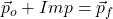Where: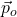,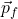– Initial and final momentums, measured in kilogram-meters per second.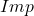– Impact due to collision, measured in kilogram-meters per second.

The impact experimented by the ball due to collision is: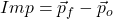By using the definition of momentum, the expression is therefore expanded: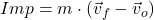Where: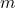– Mass of the ball, measured in kilograms.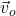,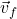– Initial and final velocities, measured in meters per second.

If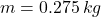,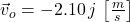and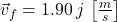, the vectorial change of the linear momentum is: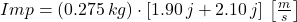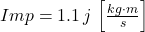The magnitude of the change in the ball’s momentum is 1.1 kilogram-meters per second.

b) The magnitudes of initial and final momentums of the ball are, respectively: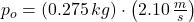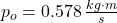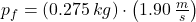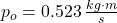The change in the magnitude of the ball’s momentum is: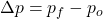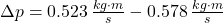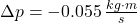The change in the magnitude of the ball’s momentum is -0.055 kilogram-meters per second.

c) The quantity calculated in part a) is more related to the net force acting on the ball during its collision with the floor, since impact is the product of net force, a vector, and time, a scalar, and net force is the product of the ball’s mass and net acceleration, which creates a change on velocity.

In a nutshell, the right choice is option D.